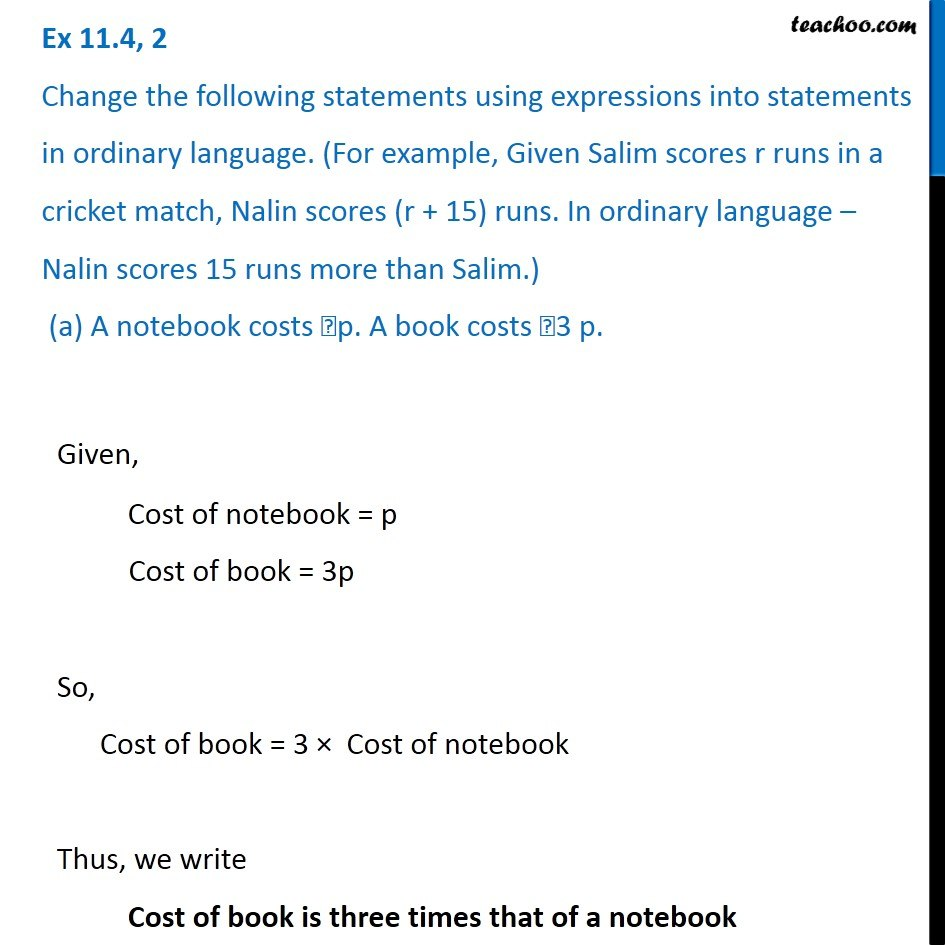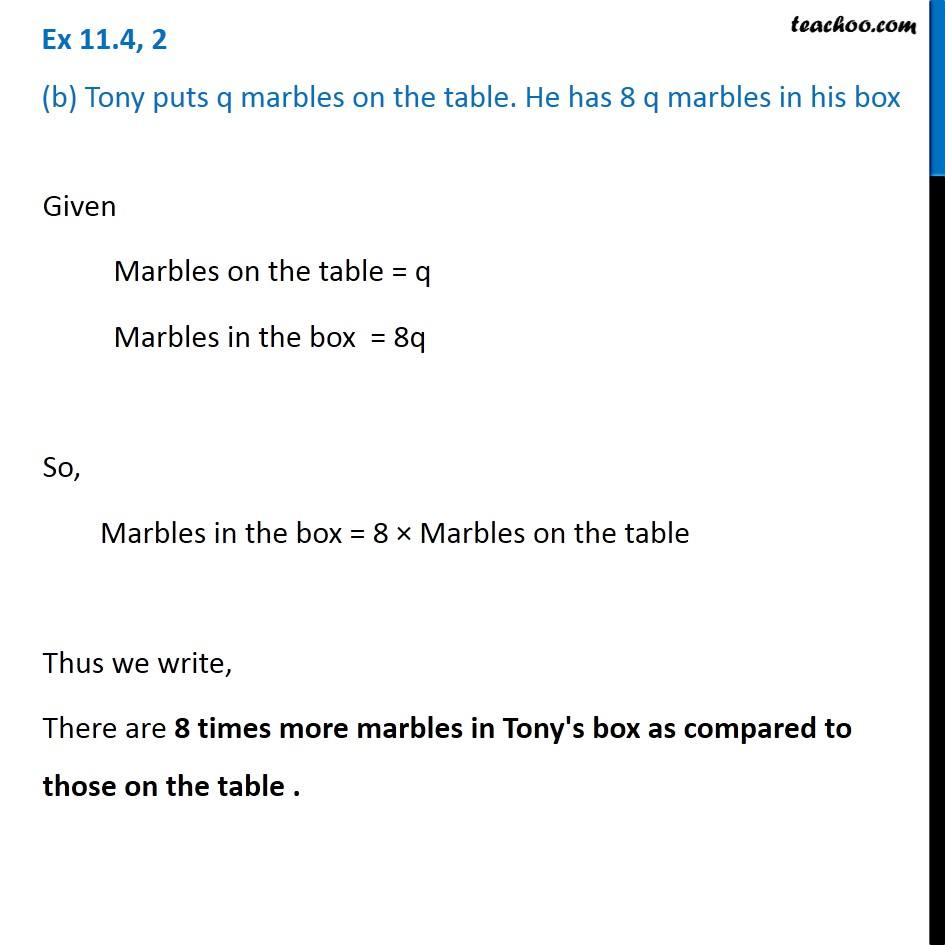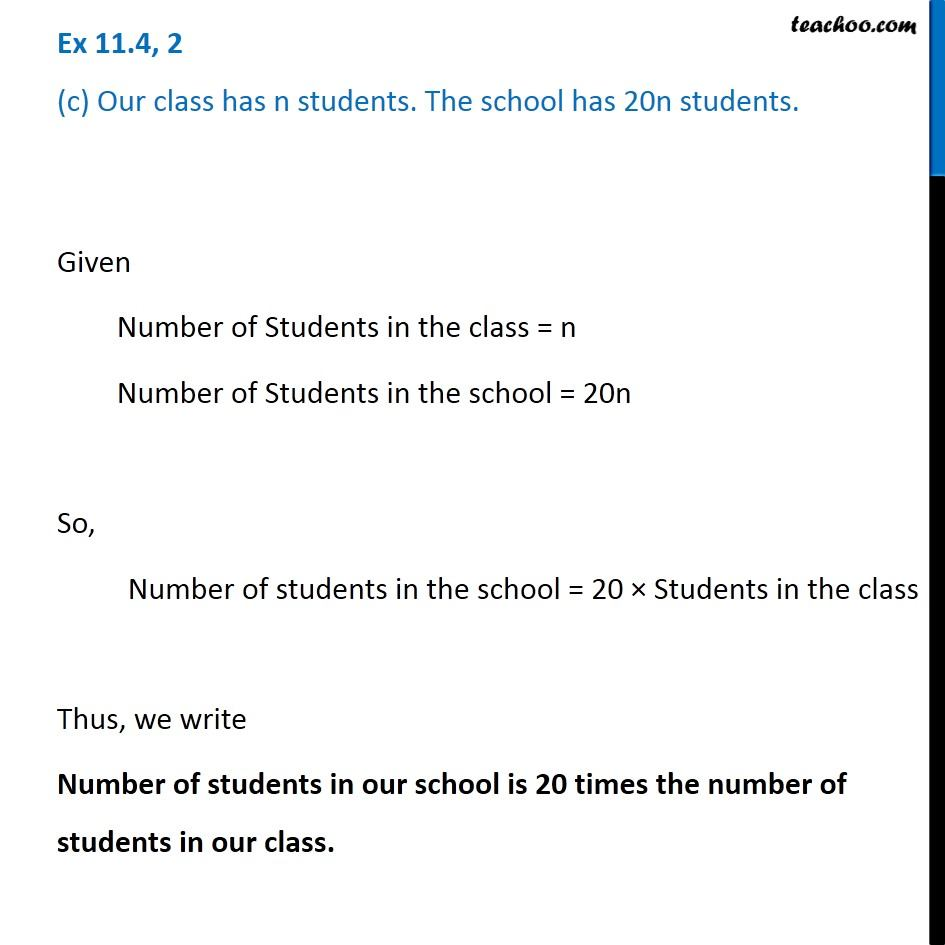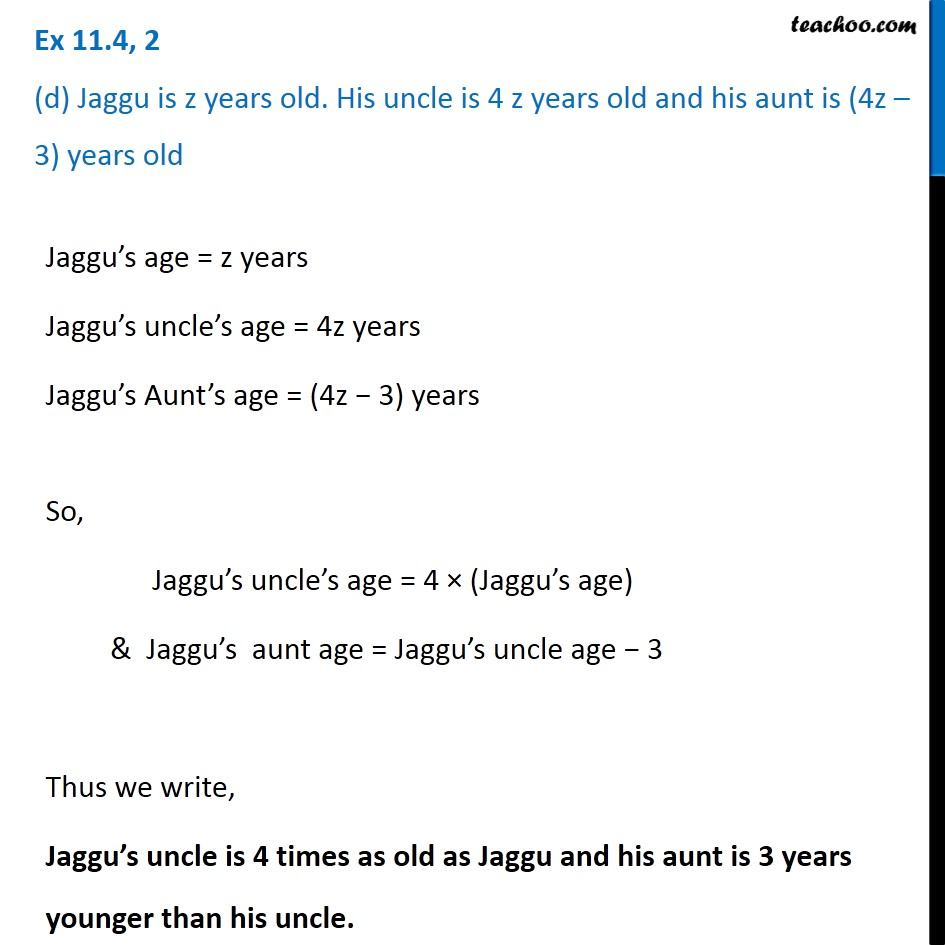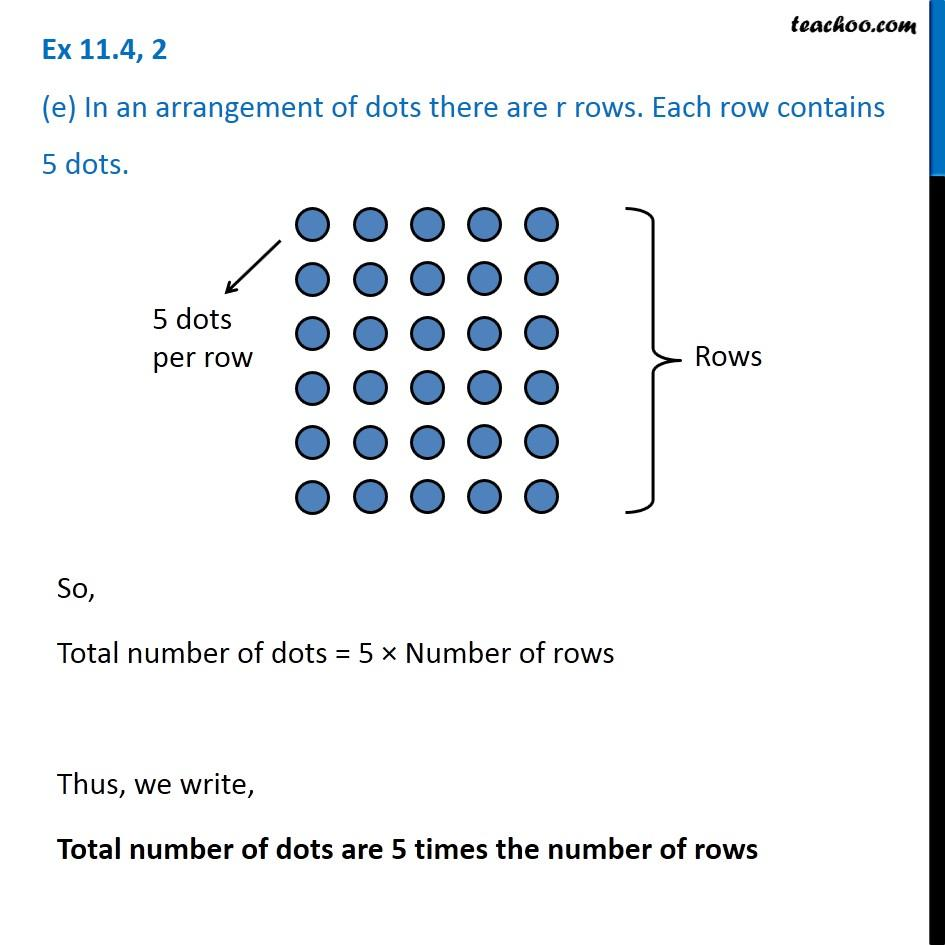Subscribe to our Youtube Channel - https://you.tube/teachoo

1. Chapter 11 Class 6 Algebra
2. Serial order wise
3. Ex 11.4

Transcript

Ex 11.4, 2 Change the following statements using expressions into statements in ordinary language. (For example, Given Salim scores r runs in a cricket match, Nalin scores (r + 15) runs. In ordinary language – Nalin scores 15 runs more than Salim.) (a) A notebook costs ₹p. A book costs ₹3 p.Given, Cost of notebook = p Cost of book = 3p So, Cost of book = 3 × Cost of notebook Thus, we write Cost of book is three times that of a notebook Ex 11.4, 2 (b) Tony puts q marbles on the table. He has 8 q marbles in his box Given Marbles on the table = q Marbles in the box = 8q So, Marbles in the box = 8 × Marbles on the table Thus we write, There are 8 times more marbles in Tony's box as compared to those on the table . Ex 11.4, 2 (c) Our class has n students. The school has 20n students. Given Number of Students in the class = n Number of Students in the school = 20n So, Number of students in the school = 20 × Students in the class Thus, we write Number of students in our school is 20 times the number of students in our class. Ex 11.4, 2 (d) Jaggu is z years old. His uncle is 4 z years old and his aunt is (4z – 3) years oldJaggu’s age = z years Jaggu’s uncle’s age = 4z years Jaggu’s Aunt’s age = (4z − 3) years So, Jaggu’s uncle’s age = 4 × (Jaggu’s age) & Jaggu’s aunt age = Jaggu’s uncle age − 3 Thus we write, Jaggu’s uncle is 4 times as old as Jaggu and his aunt is 3 years younger than his uncle. Ex 11.4, 2 (e) In an arrangement of dots there are r rows. Each row contains 5 dots.So, Total number of dots = 5 × Number of rows Thus, we write, Total number of dots are 5 times the number of rows

Ex 11.4

Chapter 11 Class 6 Algebra
Serial order wise